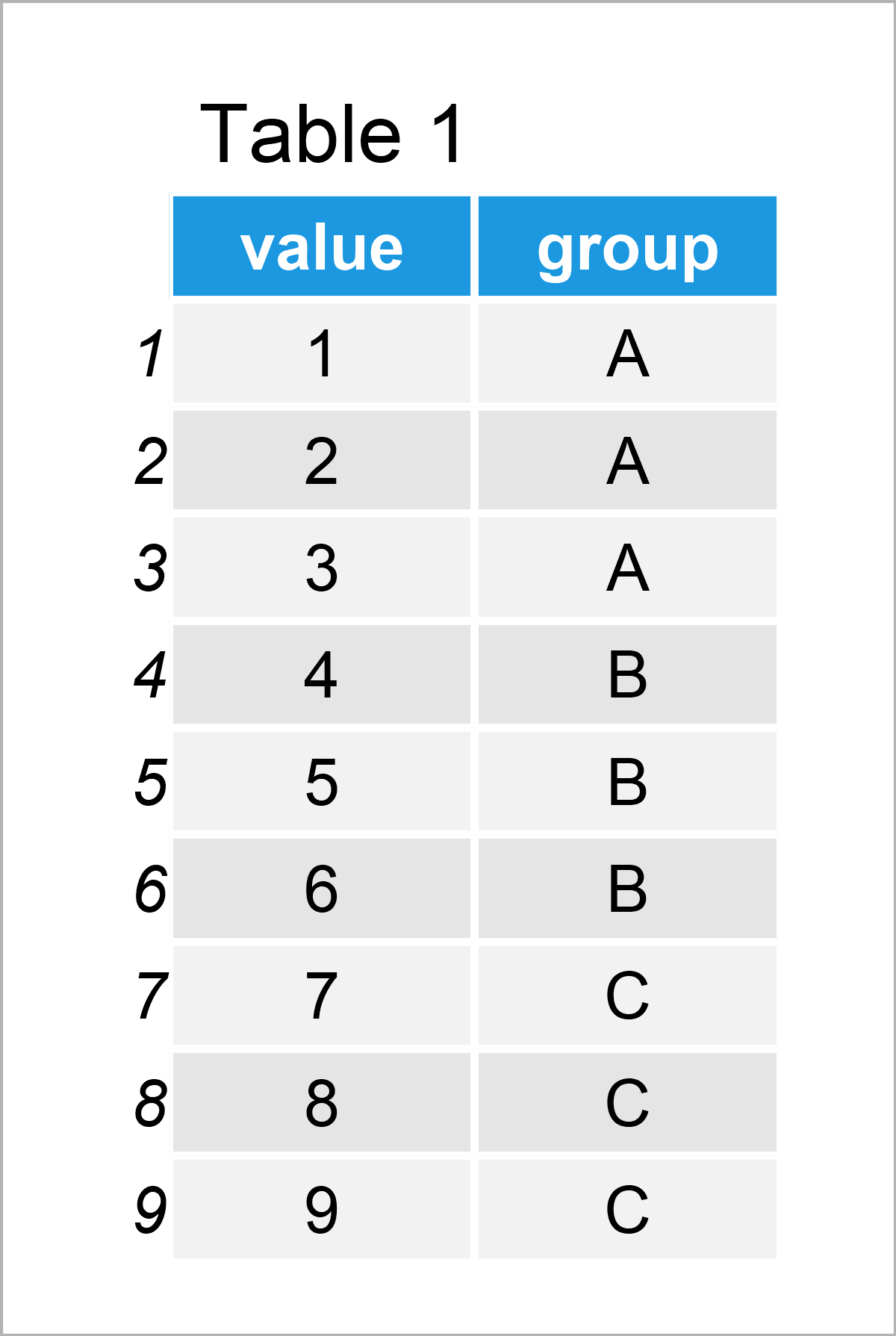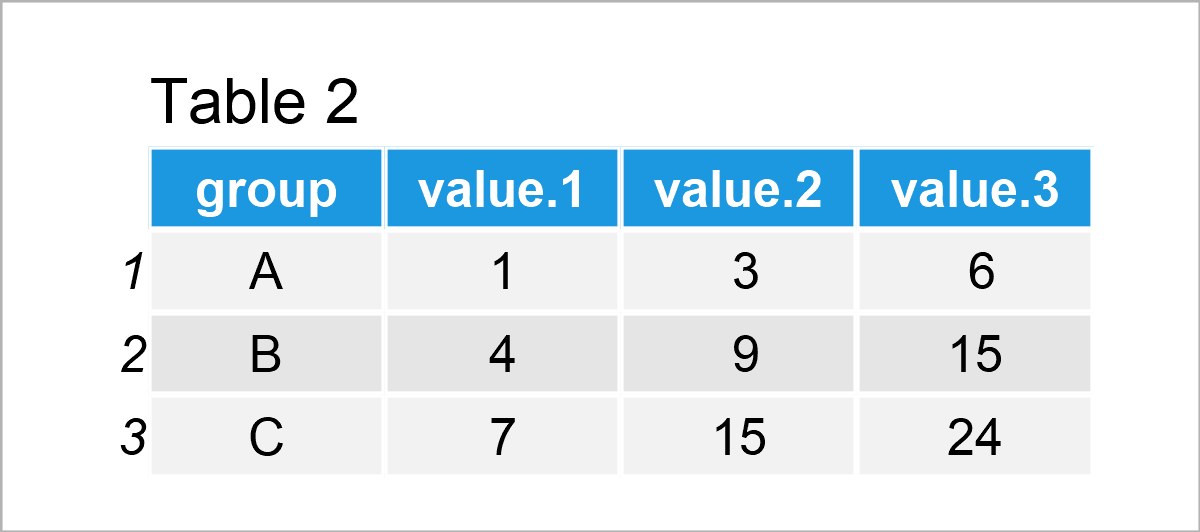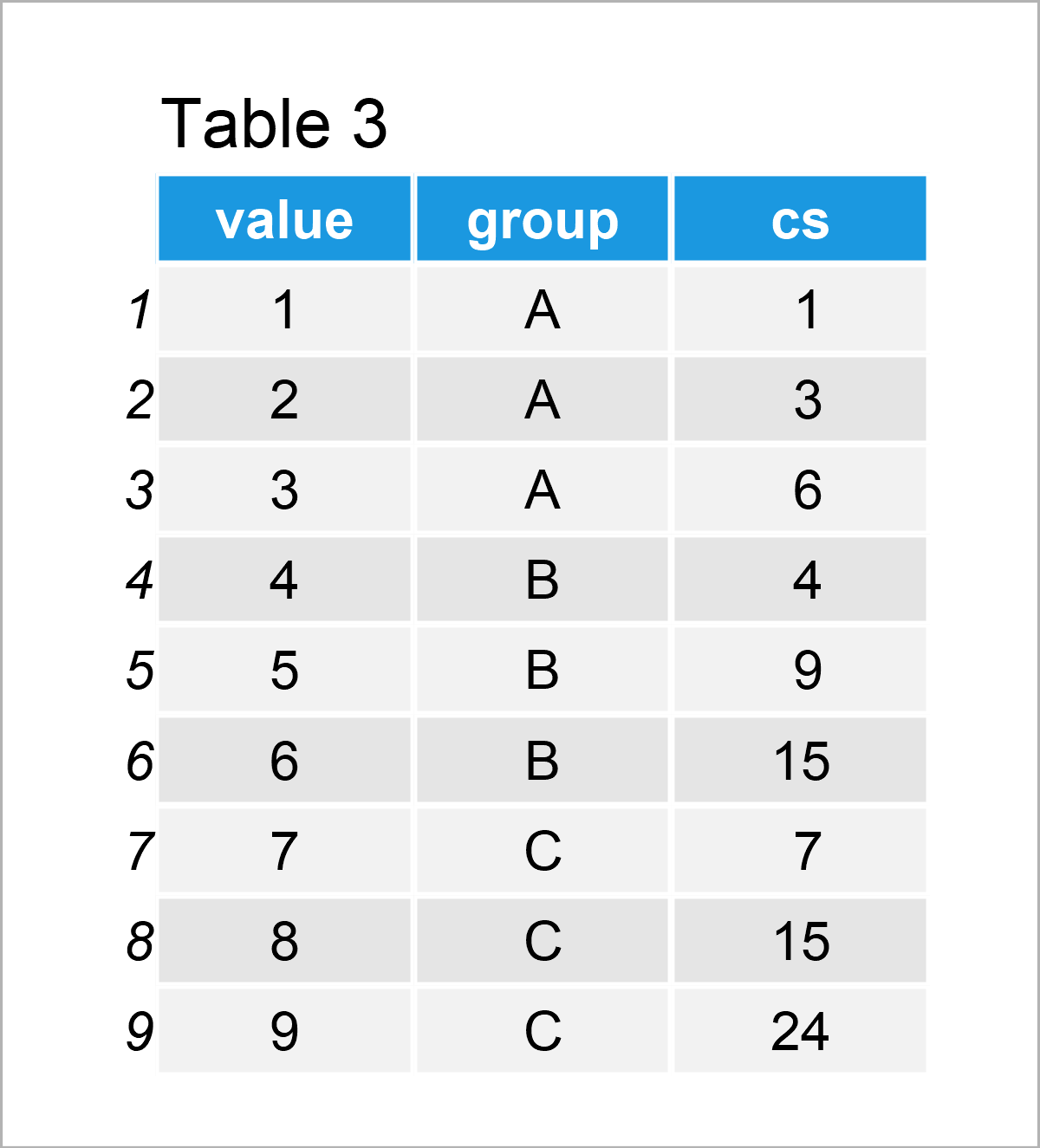# Calculate Cumulative Sum by Group in R (4 Examples)

This tutorial illustrates how to compute the cumulative sum by group in the R programming language.

The post is structured as follows:

Let’s do this!

## Creation of Example Data

I use the following data as a basis for this R tutorial:

```data <- data.frame(value = 1:9,             # Create example data frame
group = rep(LETTERS[1:3], each = 3))
data                                        # Print example data frame```Have a look at the previous table. It shows that our example data is made of nine rows and two columns. The variable value has the integer class and the variable group has the character class.

## Example 1: Create Vector of Cumulative Sums by Group Using ave() Function

In Example 1, I’ll explain how to create a vector of cumsum values by group.

For this task, we can apply the ave function as shown below:

```cs1 <- ave(data\$value,                      # Apply ave function
data\$group,
FUN = cumsum)
cs1                                         # Print vector with cumulative sum
#   1  3  6  4  9 15  7 15 24```

As you can see, we have created a new vector containing the cumulative sum by group.

## Example 2: Create Cumulative Sum Data Frame Column for Each Group Using aggregate() Function

This example shows how to create a data frame with an additional column containing the cumulative sum for each group.

To achieve this, we can apply the aggregate function as demonstrated in the following R code:

```cs2 <- aggregate(value ~ group,             # Apply aggregate function
data,
cumsum)
cs2                                         # Print data frame with cumulative sum```Note that the value.1, value.2, and value.3 columns are part of a matrix that is contained in the second column of the previously created data frame.

## Example 3: Create dplyr tibble with Cumulative Sum by Group

In Example 3, I’ll show how to use the functions of the dplyr package to get the cumulative sum by group.

We first have to install and load the dplyr package:

```install.packages("dplyr")                   # Install & load dplyr
library("dplyr")```

In the next step, we can apply the group_by and mutate functions of the dplyr package to create a new data frame column that contains the cumsum values for each group.

It is important to specify the dplyr package name in front of the mutate function, in case you have loaded the plyr package as well. Otherwise, the function of the plyr package might be use leading to a cumsum value that is not calculated by group, but for the entire data frame.

```cs3 <- data %>%                             # Apply group_by & mutate functions
group_by(group) %>%
dplyr::mutate(cs = cumsum(value))
cs3                                         # Print tibble with cumulative sum```Table 3 shows that we have added a new column to our data frame that contains the cumulative sum values by group. Note that the previous R code has created a tibble object.

## Example 4: Create data.table with Cumulative Sum by Group

In Example 4, I’ll illustrate how to return the cumulative sum by group using the data.table package.

To be able to use the functions of the data.table package, we first need to install and load data.table:

```install.packages("data.table")              # Install data.table package

Next, we can apply the setDT function to construct a new data.table column containing the cumsum values for each group:

```cs4 <- data                                 # Duplicate data frame
setDT(cs4)[, cs := cumsum(value), group]    # Apply setDT function
cs4                                         # Print data.table with cumulative sum```The output values in the previous table are the same as in the previous example. However, this time we have created a data.table object.

## Video, Further Resources & Summary

I have recently released a video on my YouTube channel, which shows the topics of this tutorial. Please find the video below.

Please accept YouTube cookies to play this video. By accepting you will be accessing content from YouTube, a service provided by an external third party.If you accept this notice, your choice will be saved and the page will refresh.

In addition, you may want to have a look at the related tutorials on statisticsglobe.com:

In this post you have learned how to calculate the cumulative sum by groups in a data frame in R programming. In case you have further questions or comments, please let me know in the comments.

Subscribe to the Statistics Globe Newsletter